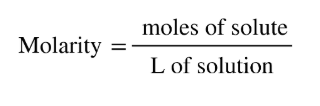# Problem: How many moles of KCl are contained in each of the following?1.7 L of a 0.86 M KCl solution

###### FREE Expert Solution
• Recall that molarity can be calculated as:97% (254 ratings)###### Problem Details

How many moles of KCl are contained in each of the following?

1.7 L of a 0.86 M KCl solution